• 利用最小二乘法可以简便地求得未知的数据，并使得这些求得的数据与实际数据之间误差的平方和为最小最小二乘法还可用于曲线拟合。其他一些优化问题也可通过最小化能量或最大化熵用最小二乘法来表达。 以最简单的...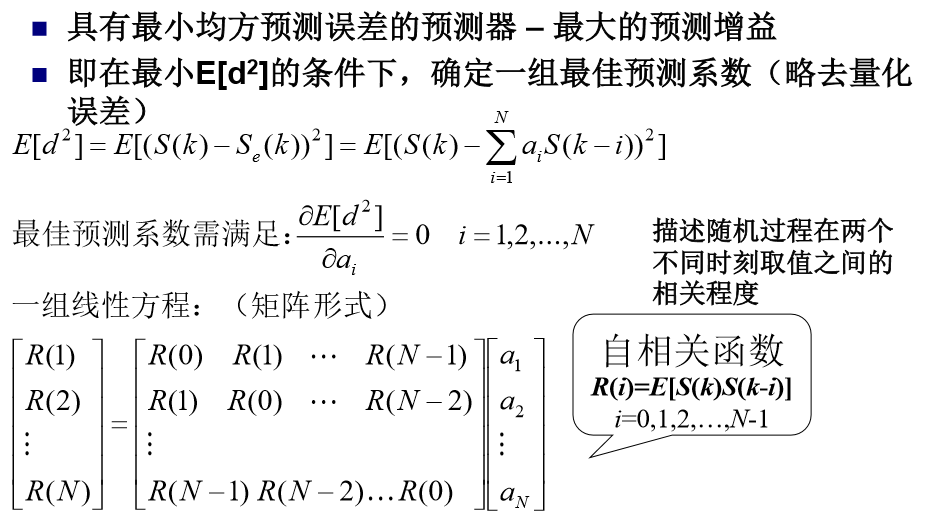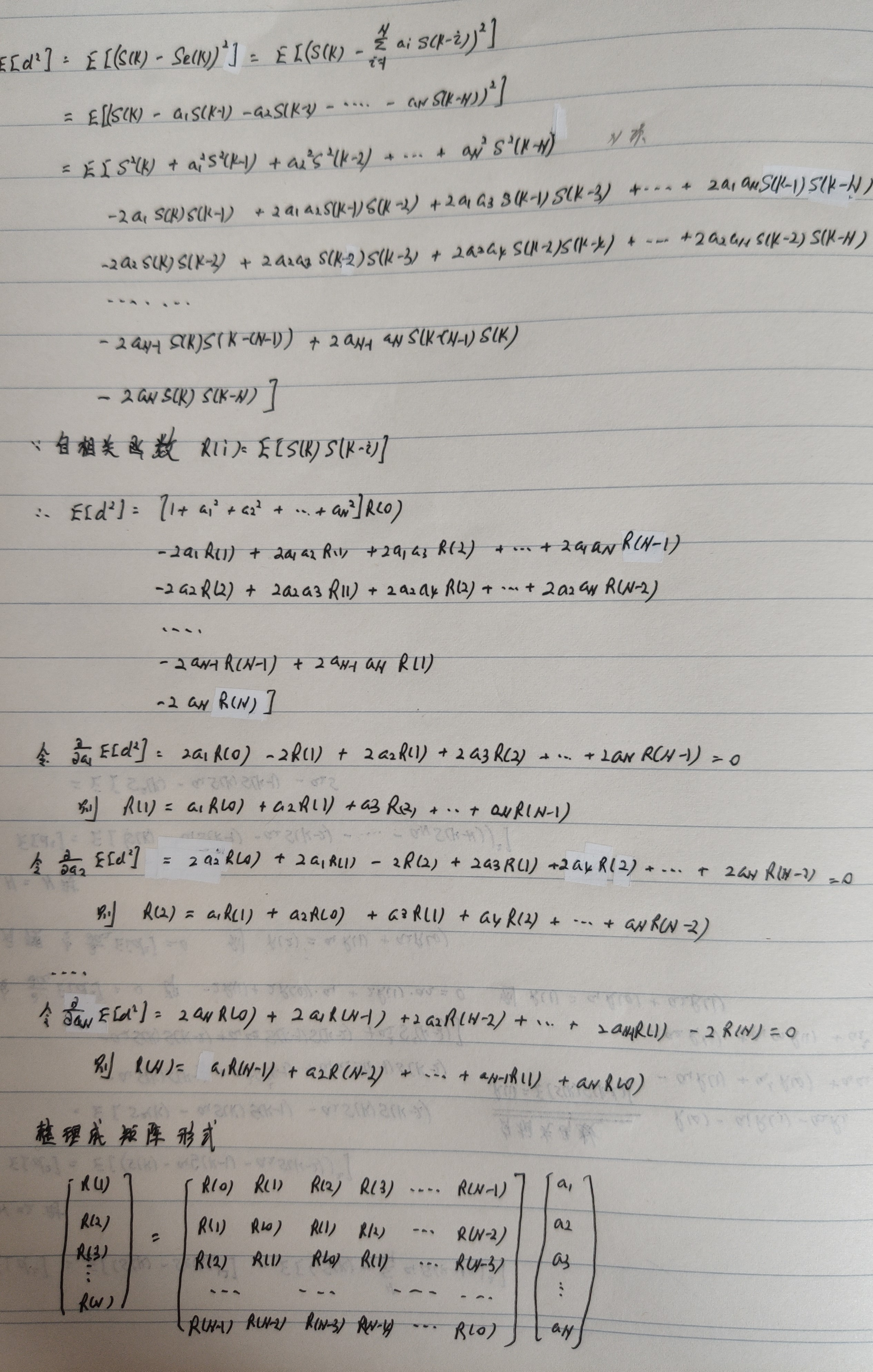最小二乘法（又称最小平方法）是一种数学优化技术。它通过最小化误差的平方和寻找数据的最佳函数匹配。利用最小二乘法可以简便地求得未知的数据，并使得这些求得的数据与实际数据之间误差的平方和为最小。最小二乘法还可用于曲线拟合。其他一些优化问题也可通过最小化能量或最大化熵用最小二乘法来表达。
基本公式
考虑超定方程组（超定指方程个数大于未知量个数）：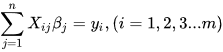其中m代表有m个等式，n代表有n个未知数β，m>n ；将其进行向量化后为：Xβ=y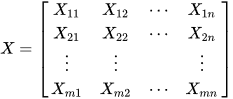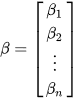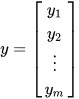显然该方程组一般而言没有解，所以为了选取最合适的β让该等式"尽量成立"，引入残差平方和函数S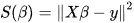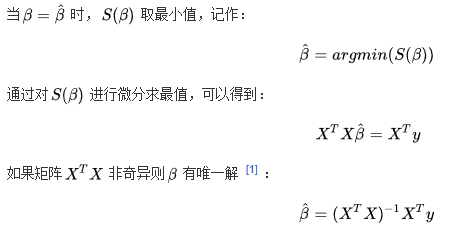方法
以最简单的一元线性模型来解释最小二乘法。什么是一元线性模型呢？监督学习中，如果预测的变量是离散的，我们称其为分类（如决策树，支持向量机等），如果预测的变量是连续的，我们称其为回归。回归分析中，如果只包括一个自变量和一个因变量，且二者的关系可用一条直线近似表示，这种回归分析称为一元线性回归分析。如果回归分析中包括两个或两个以上的自变量，且因变量和自变量之间是线性关系，则称为多元线性回归分析。对于二维空间线性是一条直线；对于三维空间线性是一个平面，对于多维空间线性是一个超平面。

对于一元线性回归模型, 假设从总体中获取了n组观察值（X1，Y1），（X2，Y2）， …，（Xn，Yn）。对于平面中的这n个点，可以使用无数条曲线来拟合。要求样本回归函数尽可能好地拟合这组值。综合起来看，这条直线处于样本数据的中心位置最合理。 选择最佳拟合曲线的标准可以确定为：使总的拟合误差（即总残差）达到最小。有以下三个标准可以选择：
（1）用“残差和最小”确定直线位置是一个途径。但很快发现计算“残差和”存在相互抵消的问题。
（2）用“残差绝对值和最小”确定直线位置也是一个途径。但绝对值的计算比较麻烦。
（3）最小二乘法的原则是以“残差平方和最小”确定直线位置。用最小二乘法除了计算比较方便外，得到的估计量还具有优良特性。这种方法对异常值非常敏感。
最常用的是普通最小二乘法（ Ordinary Least Square，OLS）：所选择的回归模型应该使所有观察值的残差平方和达到最小。（Q为残差平方和）- 即采用平方损失函数。

样本回归模型：（其中ei为样本（Xi,Yi）的误差）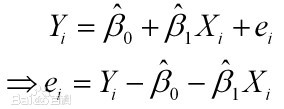平方损失函数：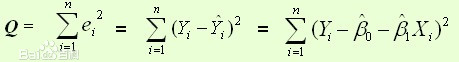则通过Q最小确定这条直线，即确定β0和β1，把它们看作是Q的函数，就变成了一个求极值的问题，可以通过求导数得到。求Q对两个待估参数的偏导数：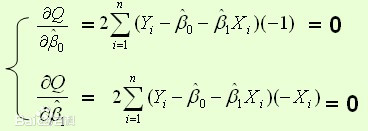根据数学知识我们知道，函数的极值点为偏导为0的点。
解得：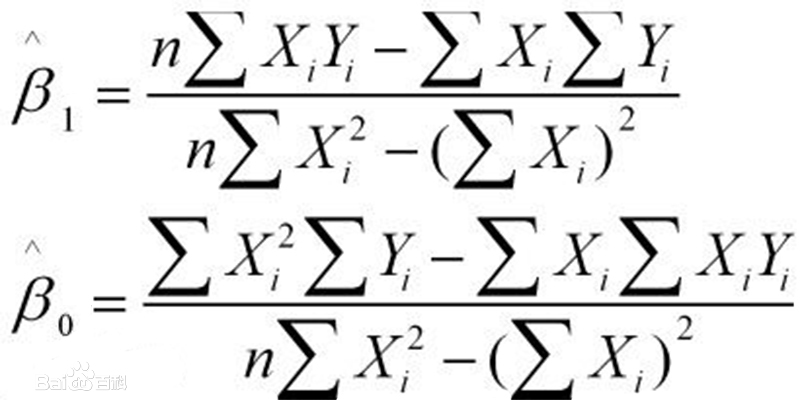这就是最小二乘法的解法，就是求得平方损失函数的极值点。

我们给出线性回归的损失函数，为了方便，不带正则项：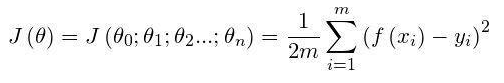其中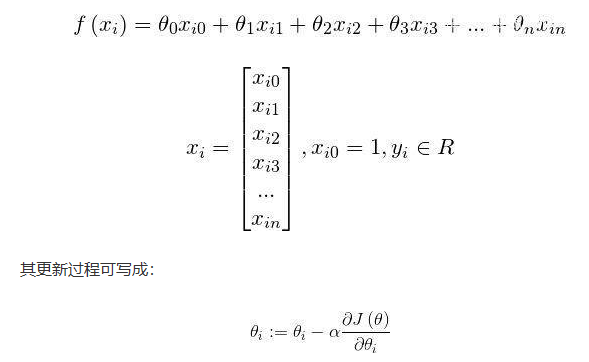具体的梯度下降流程：
第一步：先随便假设一组θ,你要是喜欢可以全部取0
第二步循环迭代:
第一次迭代：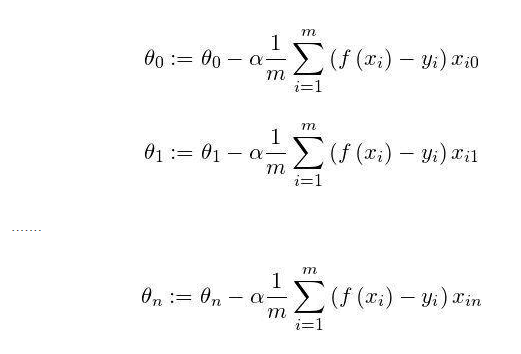第二次迭代：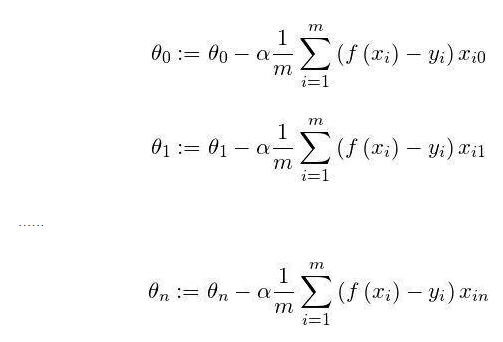第x次迭代：…
第三步，满足要求，循环结束，得到θ
最小二乘法跟梯度下降法都是通过求导来求损失函数的最小值，那它们有什么异同？
相同：
1.本质相同：两种方法都是在给定已知数据（independent & dependent variables）的前提下对dependent variables算出出一个一般性的估值函数。然后对给定新数据的dependent variables进行估算。
2.目标相同：都是在已知数据的框架内，使得估算值与实际值的总平方差尽量更小（事实上未必一定要使用平方），估算值与实际值的总平方差的公式为：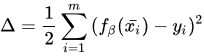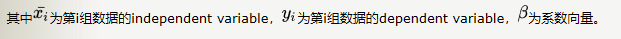不同：
1.实现方法和结果不同：最小二乘法是直接对Δ求导找出全局最小，是非迭代法。而梯度下降法是一种迭代法，先给定一个β，然后向Δ下降最快的方向调整β，在若干次迭代之后找到局部最小。梯度下降法的缺点是到最小点的时候收敛速度变慢，并且对初始点的选择极为敏感，其改进大多是在这两方面下功夫。
牛顿法
牛顿法是一种在实数域和复数域上近似求解方程的方法。方法使用函数f (x)的泰勒级数的前面几项来寻找方程f(x)=0的根。牛顿法最大的特点就在于它的收敛速度很快。
用牛顿迭代法解非线性方程，是把非线性方程f(x)=0线性化的一种近似方法。把f(x)在点x0的某邻域内展开成泰勒级数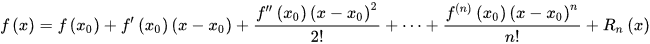取其线性部分（即泰勒展开的前两项），并令其等于0，即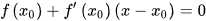以此作为非线性方程f(x)=0的近似方程，若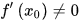则其解为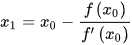得到牛顿迭代法的一个迭代关系式：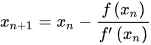已经证明，如果是连续的，并且待求的零点是孤立的，那么在零点周围存在一个区域，只要初始值位于这个邻近区域内，那么牛顿法必定收敛。 并且，如果不为0, 那么牛顿法将具有平方收敛的性能. 粗略的说，这意味着每迭代一次，牛顿法结果的有效数字将增加一倍。

高斯牛顿法
高斯一牛顿迭代法(Gauss-Newton iteration method)是非线性回归模型中求回归参数进行最小二乘的一种迭代方法，该法使用泰勒级数展开式去近似地代替非线性回归模型，然后通过多次迭代，多次修正回归系数，使回归系数不断逼近非线性回归模型的最佳回归系数，最后使原模型的残差平方和达到最小。其直观思想是先选取一个参数向量的参数值β，若函数ft(Xt，β)在β0附近有连续二阶偏导数，则在β0的邻域内可近似地将ft(Xt，β)看作是线性，因而可近似地用线性最小二乘法求解
高斯-牛顿法的一般步骤如下所示
(1) 初始值的选择。其方法有三种：
一是根据以往的经验选定初始值；
二是用分段法求出初始值；
三是对于可线性化的非线性回归模型，通过线性变换，然后施行最小平方法求出初始值
(2)泰勒级数展开式。设非线性回归模型为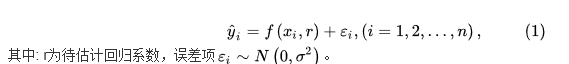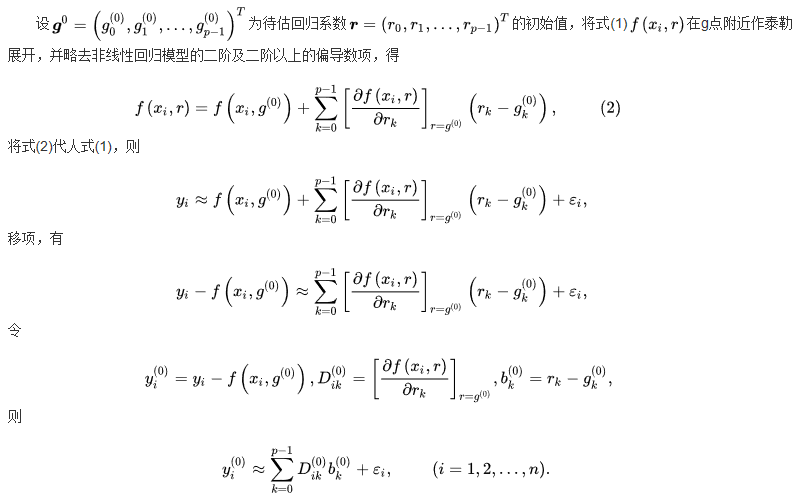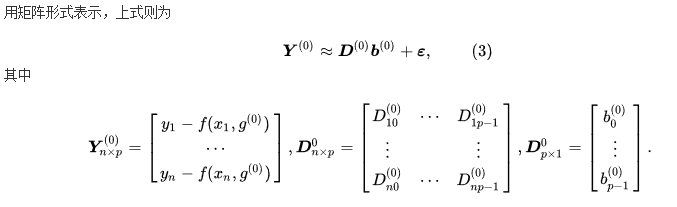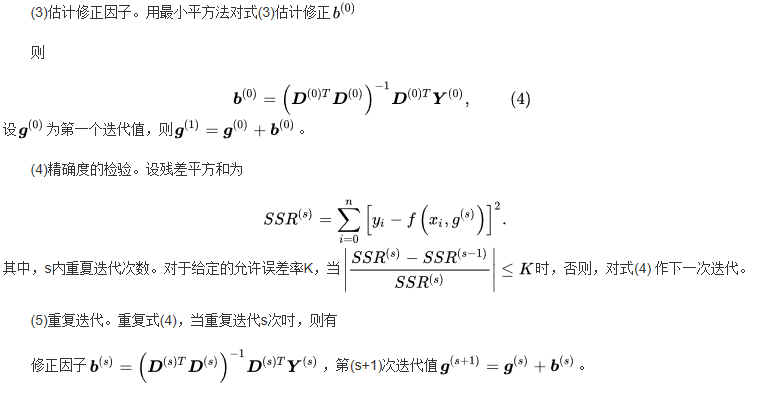最小二乘法C++实现

/*
最小二乘法C++实现
参数1为输入文件
输入 ： x
输出： 预测的y
*/
#include<iostream>
#include<fstream>
#include<vector>
using namespace std;
class LeastSquare{
double a, b;
public:
LeastSquare(const vector<double>& x, const vector<double>& y)
{
double t1=0, t2=0, t3=0, t4=0;
for(int i=0; i<x.size(); ++i)
{
t1 += x[i]*x[i];
t2 += x[i];
t3 += x[i]*y[i];
t4 += y[i];
}
a = (t3*x.size() - t2*t4) / (t1*x.size() - t2*t2);  // 求得β1
b = (t1*t4 - t2*t3) / (t1*x.size() - t2*t2);        // 求得β2
}
double getY(const double x) const
{
return a*x + b;
}
void print() const
{
cout<<"y = "<<a<<"x + "<<b<<"\n";
}
};
int main(int argc, char *argv[])
{
if(argc != 2)
{
cout<<"Usage: DataFile.txt"<<endl;
return -1;
}
else
{
vector<double> x;
ifstream in(argv);
for(double d; in>>d; )
x.push_back(d);
int sz = x.size();
vector<double> y(x.begin()+sz/2, x.end());
x.resize(sz/2);
LeastSquare ls(x, y);
ls.print();
cout<<"Input x:\n";
double x0;
while(cin>>x0)
{
cout<<"y = "<<ls.getY(x0)<<endl;
cout<<"Input x:\n";
}
}
}

参考：
百度百科
https://www.cnblogs.com/iamccme/archive/2013/05/15/3080737.html
https://baijiahao.baidu.com/s?id=1639202882632470513&wfr=spider&for=pc

展开全文• 推导如下 令 A=t−ΦwA=t-\Phi wA=t−Φw dA=t−Φdw=−ΦdwdA=t-\Phi dw=-\Phi dwdA=t−Φdw=−Φdw f=ATRAf=A^TRAf=ATRA df=(dA)TRA+ATRdA df=(dA)^TRA+A^TRdA df=(dA)TRA+ATRdAdf=tr((dA)TRA+ATRdA) df=tr((dA)^...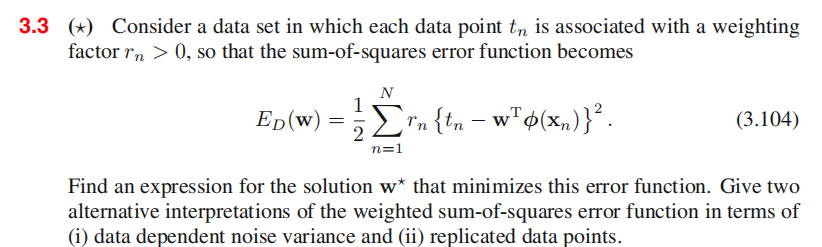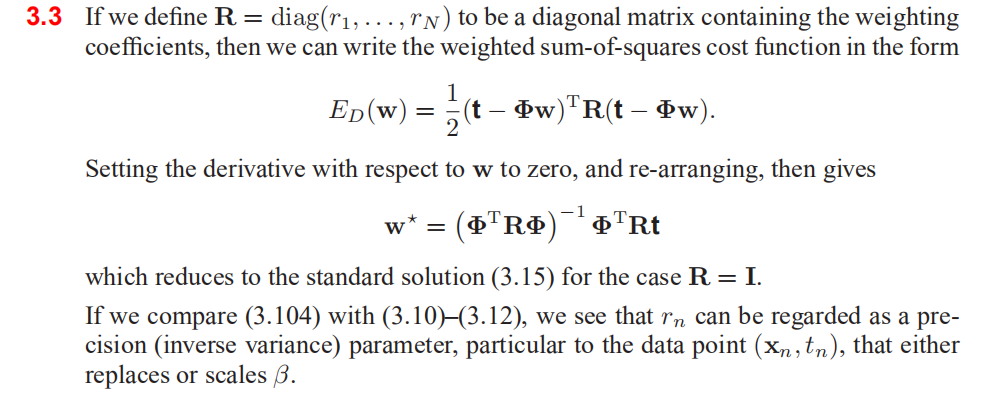推导如下

A = t − Φ w A=t-\Phi w
d A = t − Φ d w = − Φ d w dA=t-\Phi dw=-\Phi dw
f = A T R A f=A^TRA
d f = ( d A ) T R A + A T R d A df=(dA)^TRA+A^TRdA d f = t r ( ( d A ) T R A + A T R d A ) df=tr((dA)^TRA+A^TRdA) d f = t r ( A T R T d A + A T R d A ) df=tr(A^TR^TdA+A^TRdA) = t r ( A T ( R T + R ) d A ) =tr(A^T(R^T+R)dA) = t r ( 2 A T R d A ) =tr(2A^TRdA) d f = − t r ( 2 A T R Φ d w ) df=-tr(2A^TR\Phi dw)
由于 d f = （ ( ∂ f ∂ w ) T d w ） df=（( \frac{\partial f}{\partial w} )^Tdw ）
∂ f ∂ w = Φ T R T A \frac{\partial f}{\partial w}=\Phi^TR^TA = Φ T R T ( t − Φ w ) =\Phi^TR^T(t-\Phi w) = Φ T R ( t − Φ w ) =\Phi^TR(t-\Phi w)
∂ f ∂ w = = 0 \frac{\partial f}{\partial w}==0 Φ T R t = Φ T R Φ w \Phi ^TRt=\Phi^TR\Phi w w ∗ = ( Φ T R Φ ) − 1 Φ T R t w^{*}=(\Phi^TR\Phi)^{-1}\Phi^T Rt

展开全文• 可以与之前的dsp.LMSFilter作为对比，从原理来实现自适应滤波器中的最小均方误差算法（Least Mean Square） 注意：本程序仅兼容2018a以后版本 这个程序有一个地方目前还存在一些问题，就是求取自相关函数R时所用到的...

可以与之前的dsp.LMSFilter作为对比，从原理来实现自适应滤波器中的最小均方误差算法（Least Mean Square）

注意：本程序仅兼容2018a以后版本

这个程序有一个地方目前还存在一些问题，就是求取自相关函数R时所用到的方法，我通过尝试自行编写自相关函数求取方程和matlab自带的corrmtx函数作为对比，发现数值有所不同，应该是matlab自带的corrmtx函数和书本上的求解方式并不相同，所以使用时需要自行判断使用哪种方式。

clc
clear
%%构建信号%%
N=256;%信号长度
for j=1:50  %进行50次lms运算
t=1:N;
d=sin(0.1*pi*t);
figure(1)
plot(t,d);
axis([0,N,-2,2]);
%%加噪声%%
x=awgn(d,20);%awgn 加性高斯白噪声  信噪比20DB

% 一种简单的计算自相关矩阵方法
% R=x'*x;%计算自相关矩阵

%%直接计算自相关矩阵 设自相关矩阵长度为L自己写
% L=256;%自相关长度
% Rxx=zeros(L,L);
% for m=1:L
%     for n=1:L
%         mm=abs(m-n);
%         temp=0;
%         for nn=1:N-mm
%             temp=temp+x(nn)*x(nn+mm);
%         end
%         Rxx(m,n)=temp/(N-mm);
%     end
% end
% [V,D]=eig(Rxx);%特征值分解求u
% ma=max(max(D));
% u=1/ma;

% 一维实值信号x的自相关矩阵Rxx应为实对称的toeplitz矩阵，
%而一维实值信号x,y的互相关矩阵Rxy为非对称的toeplitz阵，
%matlab提供的corrmtx函数产生的并非通常意义下的autocorrelation matrix

m= 255;% dfine the time lag m+1,and m+1<=n
[rx,r]=corrmtx(x,m);%corrmtx为自相关矩阵
R=rx'*rx;
[V,D]=eig(R);%提取自相关矩阵的特征值对角阵D
maxlamuda=max(D,[],'all');%提取自相关矩阵最大特征值
u=1/maxlamuda-0.01;%保证LMS算法收敛并保持稳定的条件

%%LMS迭代滤波%%
T=50;
k=25;
p=zeros(T,N-k);%50次 误差矩阵
e=zeros(1,N);%误差矩阵
w=zeros(1,k);%滤波器长度系数
y=zeros(1,N);%滤波输出
y(1:k)=x(1:k);%假设滤波器前25点为输入值
for i=(k+1):N%开始迭代更新参数
Xn=x(i:-1:(i-k+1));%输入
y(i)=w*Xn';%输出
e(i)=d(i)-y(i);%误差
w=w+2*u*e(i)*Xn;%权值更新
end
p(j,:)=(e(k+1:N)).^2;%误差信号功率
end
for i=1:N-k
E(i)=sum(p(:,i))/T;%50次误差取平均
end
figure(2)
t=1:N-k;
plot(t,E);

仅供参考，严禁转载，感谢。

算法工作模式

参数

• 抽头权系数向量w(n)
• 输入向量x(n)
• 期望输出d(n)
• 滤波器输出y(n)
• 滤波器抽头权更新参数w(n+1)

流程

• 滤波： y ( n ) = ω T ( n ) x ( n ) \displaystyle y(n)={{\omega }^{T}}(n)x(n)
• 误差估计： e ( n ) = d ( n ) − y ( n ) \displaystyle e(n)=d(n)-y(n)
• 抽头权向量更新： ω ( n + 1 ) = ω ( n ) + 2 μ e ( n ) x ( n ) \displaystyle \omega (n+1)=\omega (n)+2\mu e(n)x(n)

注意

• 为了保证算法的收敛和稳定性必须有： 0 < μ < 1 λ max ⁡ \displaystyle 0<\mu <\frac{1}{{{{\lambda }_{{\max }}}}}
• λ max ⁡ \displaystyle {{\lambda }_{{\max }}} 为输入自相关矩阵R的最大特征值（公式证明，但实际使用时可能会出现问题，通常不这么求最大步长，但测试可以使用 1

1. 自适应滤波器原理及Matlab仿真应用（原书第2版） ↩︎

展开全文matlab 算法
• 线性最小均方误差仿真

千次阅读 2016-04-15 17:24:35
• 本文详细的介绍了如何利用线性代数的知识建模并解决一个以均方误差为损失函数的回归问题机器学习 回归 人工智能
• 均方误差准则(MSE)和LMS算法5.5.2均方误差准则(MSE)和LMS算法引言：均方误差准则同时考虑ISI及噪声的影响，使其最小化。本节讨论问题：均方误差准则；无限长LMS均衡器(C(z)，Jmin)；有限长LMS均衡器(Copt，Jmin)；...
• 准则 采用一种分类形式后，就要采用准则来衡量分类的...分类器设计准则：FIsher准则、感知机准则、最小二乘（最小均方误差）准则 Fisher准则 Fisher线性判别分析LDA（LinearityDistinctionAnalysis）基本思想：对...
• 线性最小方差估计理论推导

千次阅读 2020-04-26 23:43:24
也叫线性最小均方误差估计，顾名思义，就是使估计误差的平方和的均值达到最小。 理论推导 假设系统z=Hx+ϵz=Hx+\epsilonz=Hx+ϵ已知,xxx为真实值，zzz为观测到的数据，我们需要利用观测到的数据去估计真值，故被...统计学 最小均方误差 矩阵 机器学习
• 最小平方误差算法实验原理最小平方误差算法步骤： （1）根据N个分属于两类的样本ω1，ω2 ，写成增广向量形式，将属于ω2的训练样本乘以（-1），写出规范化增广样本矩阵X。 （2）写出X的为逆矩阵： （3）设置...python 算法 python 人工智能
• 最小二乘法的证明过程

千次阅读 2019-05-02 16:01:55
最小二乘法的证明过程 背景 在统计学中，回归分析（regression analysis）指的是确定两种或两种以上变量间相互依赖的定量关系的一种统计分析方法。在监督学习中，如果预测的变量是离散的，我们称其为分类（如决策树...
•  最小平方误差（又叫最小二乘误差）判别是针对样本线性不可分的情况来讨论的，因此当样本不可分时，就有可能出现错分，就不可能所有样本都满足，对于这种问题，我们有一个约定，就是求解一个解向量使得出现错分的...
• 感知器算法只是当被分模式可用一个特定的判别界面分开时才收敛，在不可分情况下，只要计算程序不终止，它就始终不收敛。 即使在模式可分的情况下，也很难事先算出达到收敛时所需要的迭代次数。...最小平方误差机器学习 模式识别 算法 数据 函数
• 目录PCA介绍PCA大致思路PCA人脸识别（特征脸法）matlab代码实现Python代码实现PCA几何解释PCA证明最小重构误差和最大散度等价实验结果 PCA介绍        主成分分析（Principal ...计算机视觉 机器学习 人工智能 视觉检测 python
• 最小平方误差(LMSE)算法 相对复杂，需要求逆（维数高时求逆比较困难），但对两类情况，提供了线性可分的测试特征。模式类别可分性的判别： (i) 若e(k)=0，即Xw(k)=b(k)>0，有解。 (ii) 若e(k)>0，此时隐含 ...
• 目录 1 最小二乘法简介 2 最小二乘法历史 3 基本形式 4 一元线性回归 ...利用最小二乘法可以简便地求得未知的数据，并使得这些求得的数据与实际数据之间误差的平方和为最小最小二乘法还可用于...最小二乘法 线性回归
• 现代数字信号处理复习笔记

千次阅读 2020-12-25 20:35:28
2.均方值与方差 3.随机序列的相关函数和协方差函数 1.2.3平稳随机序列及其数字特征 1.狭义（严）平稳随机序列：指其N维概率分布函数或N维概率密度函数与时间n的起始位置无关。也即平稳随机序列的
• 最小二乘自适应滤波引 言基于最小均方误差(MMSE)准则的算法, 如最陡下降法、...(002)014 【摘要】介绍了图像退化模型和约束最小二乘滤波器以及平滑约束最小二乘滤 波器,并用 MATLAB7.0 实现约束最小二乘滤波恢复图像...
• （在统计学中，残差平方和函数可以看成n倍的均方误差MSE） 梯度下降法 梯度下降法是一个最优化算法，常用于机器学习和人工智能当中用来递归性地逼近最小偏差模型。 顾名思义，梯度下降法的计算过程就是沿梯度下降的...
•  主分量分析是一种无监督学习方法，主分量是指向数据能量分布最大的轴线方向，因此可以从最小均方误差意义下对数据进行最优的表达。但是就分类任务而言，由主分量分析得到的特征却不能保证可以将各个类别最好地区...自动人脸识别基本原理
• 第一部分，结合例子，从最小均方误差的角度，直观地介绍卡尔曼滤波的原理，并给出较为详细的数学推导。 第二部分，通过两个例子给出卡尔曼滤波的实际应用。其中将详细介绍一个匀加速模型，并直观的对比系统状态模型...
• 通过最小误差的平方和寻找数据的最佳函数匹配。利用最下二乘法可以简便地求得未知数据，并使得这些求得的数据与实际数据之间误差的平方和为最小最小二乘法还可用于曲线拟合。其他一些优化问题也可以通过最小化...
• 针对该问题有如下方式求解(使用均方误差最小化): 最小二乘函数求导，让导函数为0时的结果，就是最小二乘的解。求导过程如下: 变为矩阵表达式 变为矩阵函数 对函数求偏导, 最终得到问题的解 代码...梯度下降 损失函数 均方误差
• 通过寻找在最小均方误差（Mean Square Error，MSE）下的最具代表性的投影向量，再用这些向量来表示数据。 Fisher判别分析的基本思想：利用已知类别的样本建立判别模型，对未知类别的样本进行分类。在最小均方误差...LDA 线性判别分析 机器学习
• RBF网络需要学习的参数有3个：基函数的中心ci，方差σi以及隐含层与输出层间的权值Wi，根据径向基函数中心选取方法的不同，最常见的学习方法有：自组织选取中心法、正交最小二乘法等方法。 自组织学习过程中确定ci...算法 机器学习
• 输出：根据最小二乘法原理以及各点坐标求出拟合曲线 。3.程序流程：输入已知点的个数；分别输入已知点的 X 坐标；分别输入已知点的 Y 坐标；通过调用函数，求出拟合曲线。最小二乘法原理如下：根据一组给定的实验...
• 最小二乘法

2017-05-18 10:34:00
高斯于1823年在误差e1 ,… , en独立同分布的假定下,证明最小二乘方法的一个最优性质: 在所有无偏的线性估计类中,最小二乘方法是其中方差最小的！在德国10马克的钞票上有高斯像，并配了一条正态曲线。在高斯众多...
• ... 1.1 线性回归 1.1.1 原理简介 线性回归是对一次函数的拟合y=θ1x1+……+θixi+b，其中xi为自变量，每一个输入向量（x1……xi...设定损失函数，一般为误差均方根（RMSE），或者直接用均方误差，对参数求偏导数，...
• 输出：根据最小二乘法原理以及各点坐标求出拟合曲线 。3.程序流程：(1)输入已知点的个数；(2)分别输入已知点的X坐标；(3)分别输入已知点的Y坐标；(4)通过调用函数，求出拟合曲线。最小二乘法原理如下：根据一组给定......